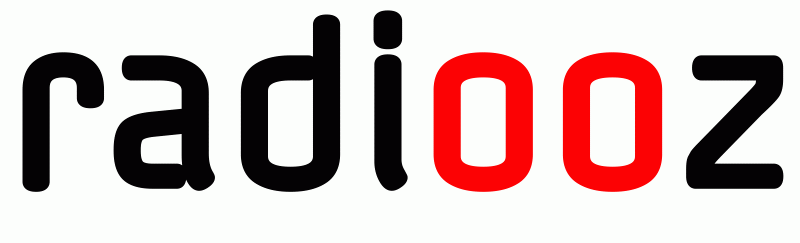S T R E A M I N G   C O N T E N T   P R O V I D E R

A L L I A N C E   F O R   E X C H A N G I N G   K N O W L E D G E   &   E X P E R I E N C E